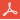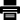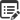# Math Functions### 1.Quick Glance#Although JavaScript comes equipped with plenty of Math Functions(w3schools), GPE adds on top of those an array of functions for basic math that may be useful for game development.

Yes NoSuggest edit

### 2.Math Functions#

#### float GPE.abs( float x)

Returns absolute value of the number x. Returns 0 if invalid datatype is entered.

Scope: GPE

Return type : float

Parameters:

• float x - the number to change

#### float GPE.bound_number( float x, float min, float max)

Returns the number bounded by min and max. If the number is between min and max it returns itself.

Scope: GPE

Return type : float

Parameters:

• float x - the number to change
• float min - the lowest allowed value to return
• float max - the highest allowed value to return.

Returns the number radians converted to degrees.

Scope: GPE

Return type : float

Parameters:

• float radians - the number to change to degrees

#### float GPE.get_direction( float x1, float y1, float x2, float y2)

Returns the calculated angle found from point (x1,y1) to point(x2, y2).

Scope: GPE

Return type : float

Parameters:

• float x1 - the x-coordinate of the first point
• float y1 - the y-coordinate of the first point
• float x2 - the x-coordinate of the second point
• float y2 - the y-coordinate of the second point

#### float GPE.get_distance( float x1, float y1, float x2, float y2)

Returns the calculated distance found from point (x1,y1) to point(x2, y2).

Scope: GPE

Return type : float

Parameters:

• float x1 - the x-coordinate of the first point
• float y1 - the y-coordinate of the first point
• float x2 - the x-coordinate of the second point
• float y2 - the y-coordinate of the second point

#### intGPE.get_random( int min, int max)

Returns a random integer between the min and max values.

Scope: GPE

Return type : int

Parameters:

• float min - the minimum random number to return.
• float max- the maximum random number to return.

#### int GPE.round( float x)

Rounds the number x.

Scope: GPE

Return type : int

Parameters:

• float x - the number to change

Returns the number degrees converted to radians.

Scope: GPE

Return type : float

Parameters:

• float degreedAngles- the number to change to radians.

#### int GPE.sign( float x)

Rounds the sign of the number. Returns -1 for negative numbers. 1 for positive numbers. 0 for 0 and NaN for invalid data types.

Scope: GPE

Return type : int

Parameters:

• float x - the number whose sign is in question
Yes NoSuggest edit